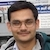# NAEST 2015 Screening Test: Cylinder Rolling Down an Inclined PlaneBy

Two cylinders of the same dimensions and equal masses are released simultaneously from the top of an incline. The surface properties of the two cylinders are identical. Both cylinders start rolling down without slipping. These cylinders reach the bottom of incline at different times. Why the cylinders take different time to reach the bottom?

Question 1: The cylinders and inclined plane are made up of non-magnetic material. The surface properties of the two cylinders are indentical. The time taken by the two cylinders to reach the ground varies with change in inclination angle. The time taken is more for larger inclination angle or smaller inclination angle?

Question 2: The yellow color cylinder takes lesser time in comparison to the time taken by the blue color cylinder. Explain in detail?

Answer: The coefficient of friction for the two cylinders is equal because they have identical surface properties.

The mass distribution in the two cylinders is different. The yellow cylinder P has most of its mass concentrated near its surface while the blue cylinder Q has most of its mass concentrated near its axis. The moment of inertia (about the cylinder axis) of the yellow cylinder is more than that of the blue cylinder i.e., $I_P > I_Q$. The difference in moment of inertia makes the cylinders to move with different accelerations.The forces acting on the cylinder are weight $mg$, normal reaction $N$, and frictional force $f$ (see figure). Let $r$ be the radius of the cylinder. The torque about centre of mass is related to the angular acceleration $\alpha$ by, \begin{align} \tau=rf=I\alpha. \end{align} The force along the inclined plane is related to the linear acceleration $a$ of the centre of mass by \begin{align} mg\sin\theta-f=ma. \end{align} In case of rolling without slipping, \begin{align} a = \alpha r. \end{align} Solve the above equations to get \begin{align} a=\frac{g\sin\theta}{1+I/(mr^2)} \end{align}

The acceleration of the centre of mass increases with the inclination angle $\theta$. Thus, the cylinder take lesser time to reach the bottom when inclination angle is increased.

The acceleration also depends on the moment of inertia. Thus $a_P < a_Q$ because $I_P > I_Q$. Hence, P reaches the ground latter, it will have lesser velocity $v$, lesser angular velocity ($\omega = v/r$) and lesser translational kinetic energy.

I encourage you to use energy method to arrive at these results. This demonstration is based on the IIT JEE 2012 problem on rotational mechanics.